# Arduino Metal Detector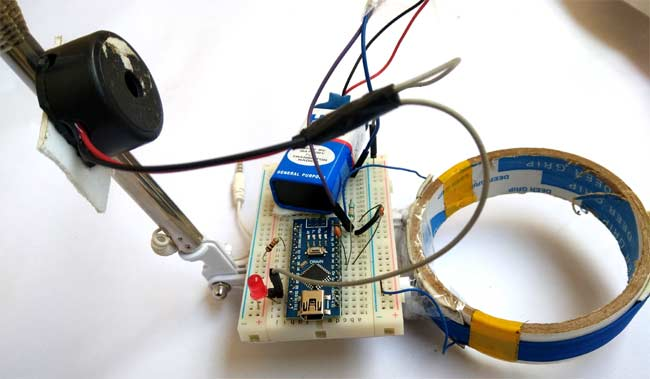A metal detector is a safety device that detects potentially harmful metals. Previously we have made very simple metal detectors without microcontroller , now we are building metal detectors using Arduino . In this project we will use coils and capacitors to detect metals. Here we have used Arduino Nano to build this metal detector project . This is a very interesting project for all electronics hobbyists. The buzzer will beep very quickly whenever this detector detects any metal nearby.

### Required components:

Here are the components you need to build a simple DIY metal detector using Arduino . All of these components should be readily available at your local hardware store.

1. Arduino (any)
2. coil
3. 10nF capacitor
4. buzzer
5. 1k resistor
6. 330 ohm resistor
7. led
8. 1N4148 Diode
10. connecting line
11. 9v battery

## How do metal detectors work?

Whenever there is current flowing through the coil, a magnetic field is created around the coil. Changes in the magnetic field create an electric field. Now according to Faraday’s law, due to this electric field, there will be a voltage across the coil that is the opposite of the change in the magnetic field , this is how the coil creates inductance , which means that the resulting voltage is the opposite of the increase in current. The unit of inductance is Henry, and the formula for measuring inductance is:

L = ( μ ο ∗ N 2 ∗ A ) / l L = (μ_ο * N^2 * A) / l
Where,
L- Inductance in Henries (inductance, in Henry)
μο- Permeability, its 4π*10 -7 for Air
N- Number of turns
A- Inner Core Area (πr 2 ) in m 2 (πr 2 ) in m 2
l- Length of the Coil in meters Coil length in meters

When any metal comes close to the coil, the coil changes its inductance. This change in inductance depends on the metal type. For non-magnetic metals it decreases and for metallic magnetic materials (like iron) it increases.

Depending on the core of the coil, the inductance value changes drastically. In the image below you can see air core inductors, in these inductors, there is no solid core . They are basically coils left in the air. The flow medium of the magnetic field produced by the inductor is empty or air. The inductance value of these inductors is very small.These inductors can be used when a few microhenries are required. Not suitable for values ​​greater than a few millihenries. In the image below you can see an inductor with a ferrite core . These ferrite core inductors have very large inductance values.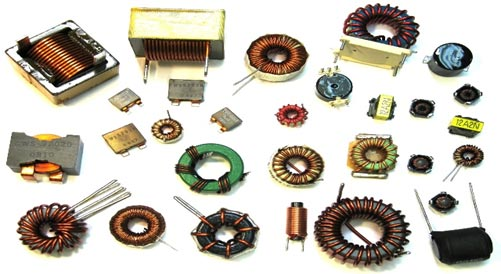Remember that the coil wound here is an air core coil, so when a piece of metal is placed near the coil, that metal piece will act as the core of the air core inductor. With this metal as the core, the inductance of the coil is changed or significantly increased. The total reactance or impedance of the LC circuit changes considerably due to the sudden increase in coil inductance compared to the case without the metal sheet.

So, in this Arduino Metal Detector project , we have to find the inductance of the coil to detect metal. For this we use the already mentioned LR circuit (resistor-inductor circuit). In this circuit, we used a coil of about 20 turns or a 10cm diameter winding. We used an empty roll of tape and wrapped the wire around it to make a coil .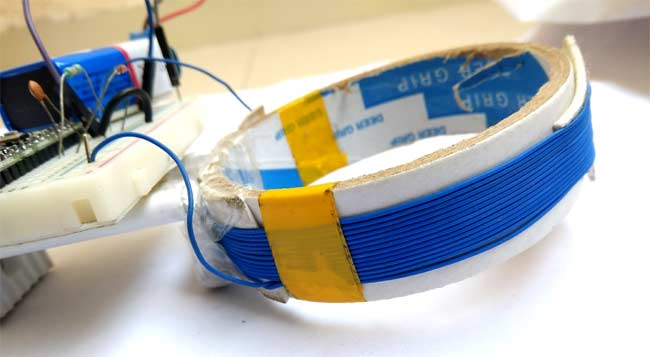## Circuit diagram:

We have used an Arduino Nano to control the entire Metal Detector project . LED and buzzer are used as metal detection indicators. Coils and capacitors are used to detect metals. Signal diodes are also used to reduce voltage. There is also a resistor to limit the current flowing to the Arduino pins.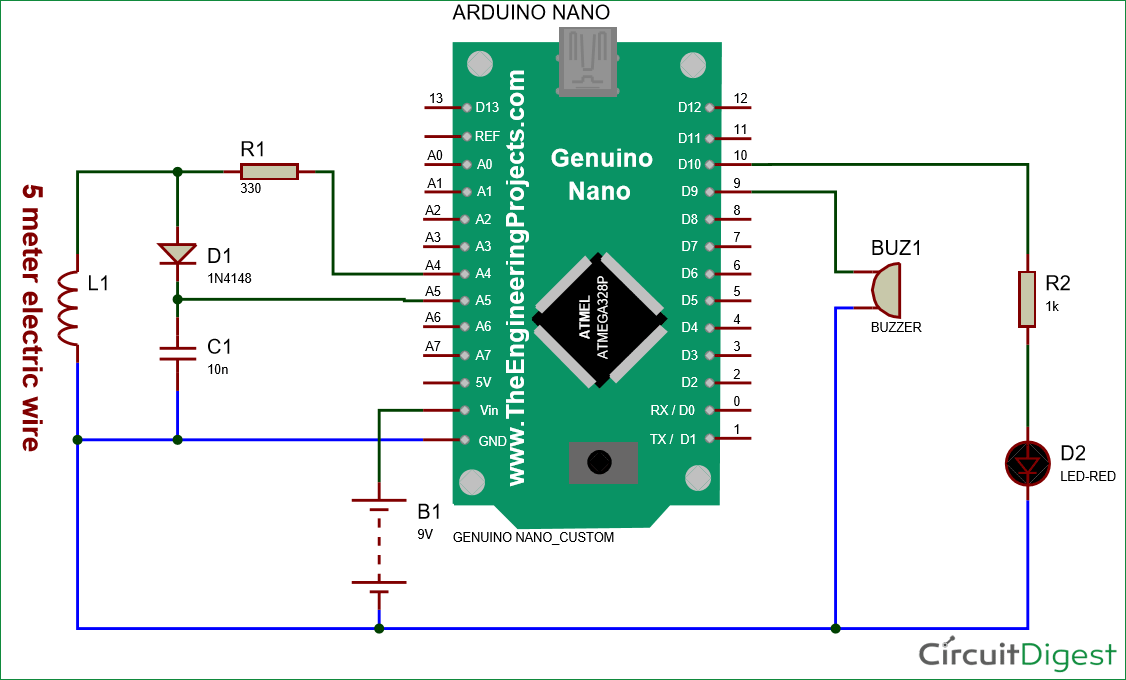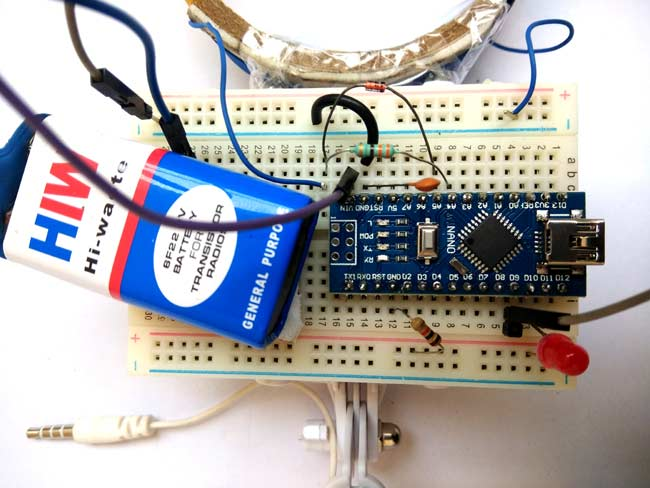## Working process Description:

Working with this Arduino Metal Detector is a little tricky. Here we feed the block wave or pulse generated by the Arduino to the LR high pass filter. Therefore, at each transition stage, the coil produces short spikes. The pulse length of the resulting spike is proportional to the inductance of the coil. So with these spikes, we can measure the inductance of the coil. But it’s hard to measure the inductance of those spikes precisely here because the duration of these spikes is very short (~0.5 microseconds) and the Arduino is difficult to measure.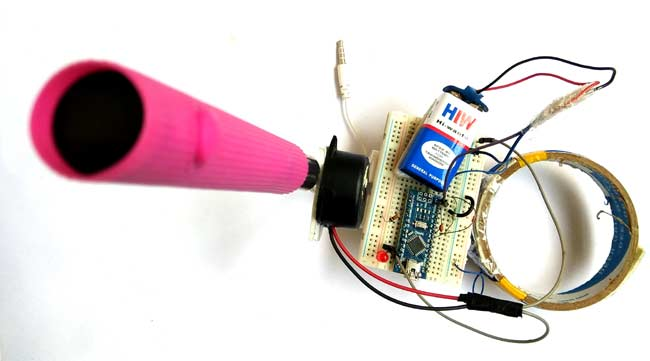So instead we use a capacitor charged by a rising pulse or spike. And it only takes a few pulses to charge the capacitor to the point where the Arduino analog pin A5 can read its voltage. The Arduino then uses the ADC to read the voltage of this capacitor. After reading the voltage, the capacitor discharges quickly by taking the capPin pin as an output and setting it low. The whole process takes about 200 microseconds. For better results, we repeated the measurement and averaged the results. This is how we can measure the approximate inductance of Coil . After getting the result, we transmit the result to the LED and buzzer to detect the presence of metal. Check out the full code given at the end of this article to see how it works.

The complete Arduino code is given at the end of this article . In the programming part of this project, we used two Arduino pins, one to generate the block wave to be fed in the Coil, and another analog pin to read the capacitor voltage. In addition to these two pins, we also used two other Arduino pins to connect the LED and buzzer.

You can view the full code and demo video of the Arduino Metal Detector below . You will see that as soon as metal is detected, the LED and buzzer will start blinking rapidly.

## code

/* 

Metal Detector Arduino Code

*/

#define capPin A5
#define buz 9
#define pulsePin A4

#define led 10

long sumExpect=0; //running sum of 64 sums
long ignor=0;   //number of ignored sums
long diff=0;        //difference between sum and avgsum
long pTime=0;
long buzPeriod=0;

void setup()
{
Serial.begin(9600);
pinMode(pulsePin, OUTPUT);
digitalWrite(pulsePin, LOW);
pinMode(capPin, INPUT);
pinMode(buz, OUTPUT);
digitalWrite(buz, LOW);
pinMode(led, OUTPUT);
}

void loop()
{
int minval=1023;
int maxval=0;
long unsigned int sum=0;
for (int i=0; i<256; i++)
{
//reset the capacitor
pinMode(capPin,OUTPUT);
digitalWrite(capPin,LOW);
delayMicroseconds(20);
pinMode(capPin,INPUT);
applyPulses();

int val = analogRead(capPin); //takes 13x8=104 microseconds
minval = min(val,minval);
maxval = max(val,maxval);
sum+=val;

long unsigned int cTime=millis();
char buzState=0;
if (cTime<pTime+10)
{
if (diff>0)
buzState=1;
else if(diff<0)
buzState=2;
}
if (cTime>pTime+buzPeriod)
{
if (diff>0)
buzState=1;
else if (diff<0)
buzState=2;
pTime=cTime;
}
if (buzPeriod>300)
buzState=0;

if (buzState==0)
{
digitalWrite(led, LOW);
noTone(buz);
}
else if (buzState==1)
{
tone(buz,2000);
digitalWrite(led, HIGH);
}

else if (buzState==2)
{
tone(buz,500);
digitalWrite(led, HIGH);
}
}

//subtract minimum and maximum value to remove spikes
sum-=minval;
sum-=maxval;

if (sumExpect==0)
sumExpect=sum<<6; //set sumExpect to expected value
long int avgsum=(sumExpect+32)>>6;
diff=sum-avgsum;
if (abs(diff)<avgsum>>10)
{
sumExpect=sumExpect+sum-avgsum;
ignor=0;
}
else
ignor++;
if (ignor>64)
{
sumExpect=sum<<6;
ignor=0;
}
if (diff==0)
buzPeriod=1000000;
else
buzPeriod=avgsum/(2*abs(diff));
}

void applyPulses()
{
for (int i=0;i<3;i++)
{
digitalWrite(pulsePin,HIGH); //take 3.5 uS
delayMicroseconds(3);
digitalWrite(pulsePin,LOW);  //take 3.5 uS
delayMicroseconds(3);
}
}

Check Also
Close